# How to Calculate and Solve for Mass of Cylindrical Shaft | Material Selection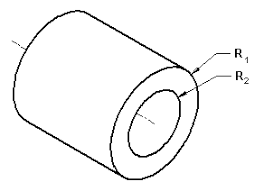The image above represents mass of cylindrical shaft.

To compute for mass of cylindrical shaft, five essential parameters are needed and these parameters are Factor of Safety (N), Twisting Moment (M), Length of Shaft (L), Density (ρ) and Shear Stress at Fracture (τf).

The formula for calculating mass of cylindrical shaft:

m = (2NM)2/31/3L)(ρ/τf2/3)

Where:

m = Mass of Cylindrical Shaft
N = Factor of Safety
M = Twisting Moment
L = Length of Shaft
ρ = Density
τf = Shear Stress at Fracture

Let’s solve an example;
Find the mass of cylindrical shaft when the factor of safety is 4, the twisting moment is 2, the length of shaft is 7, the density is 6 and the shear stress at fracture is 10.

This implies that;

N = Factor of Safety = 4
M = Twisting Moment = 2
L = Length of Shaft = 7
ρ = Density = 6
τf = Shear Stress at Fracture = 10

m = (2NM)2/31/3L)(ρ/τf2/3)
m = (2(4)(2))2/3 (π1/3(7)) (6/102/3)
m = (16)2/3 ((1.46)(7)) (6/4.64)
m = (6.349) (10.25) (1.29)
m = 84.14

Therefore, the mass of cylindrical shaft is 84.14 kg.

Nickzom Calculator – The Calculator Encyclopedia is capable of calculating the mass of cylindrical shaft.

To get the answer and workings of the mass of cylindrical shaft using the Nickzom Calculator – The Calculator Encyclopedia. First, you need to obtain the app.

You can get this app via any of these means:

To get access to the professional version via web, you need to register and subscribe for NGN 2,000 per annum to have utter access to all functionalities.
You can also try the demo version via https://www.nickzom.org/calculator

Apple (Paid) – https://itunes.apple.com/us/app/nickzom-calculator/id1331162702?mt=8
Once, you have obtained the calculator encyclopedia app, proceed to the Calculator Map, then click on Materials and Metallurgical under Engineering.Now, Click on Material Selection under Materials and MetallurgicalNow, Click on Mass of Cylindrical Shaft under Material Selection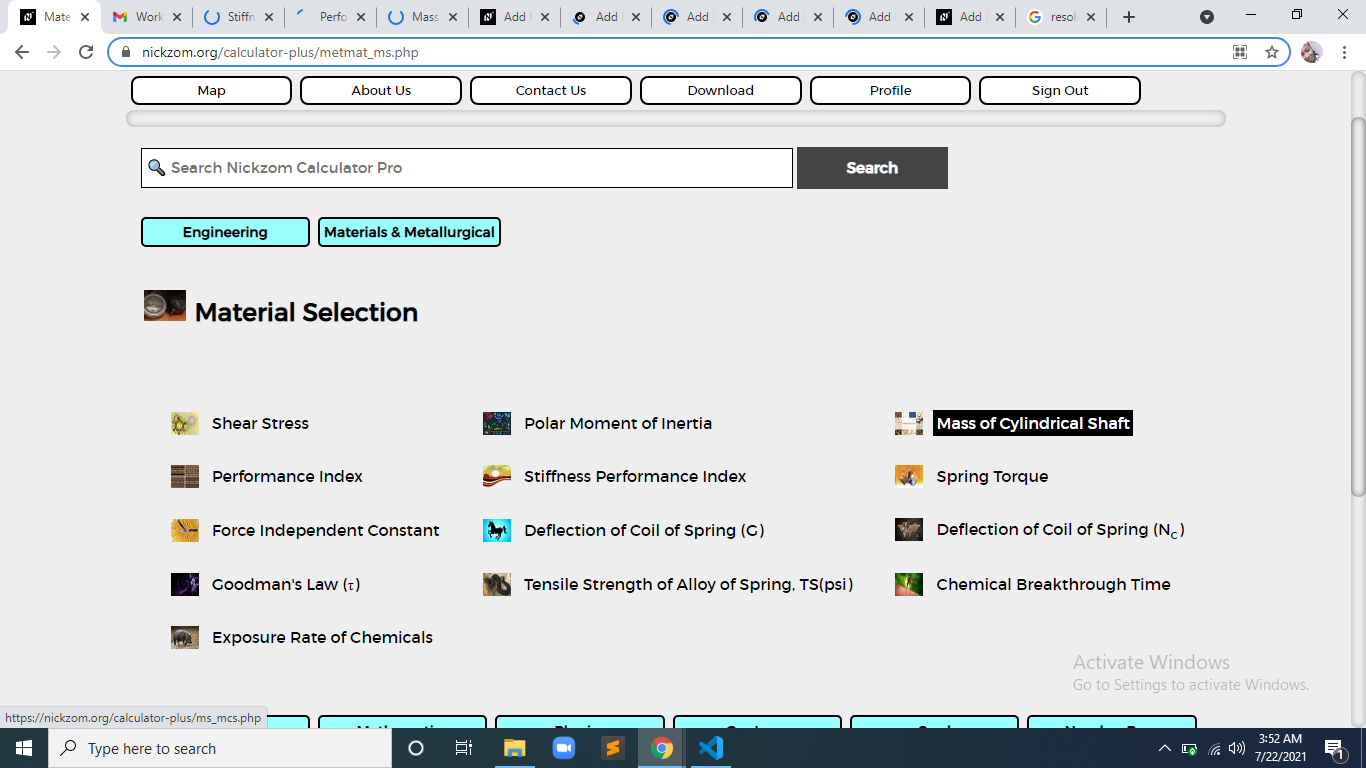The screenshot below displays the page or activity to enter your values, to get the answer for the mass of cylindrical shaft according to the respective parameter which is the Factor of Safety (N), Twisting Moment (M), Length of Shaft (L), Density (ρ) and Shear Stress at Fracture (τf).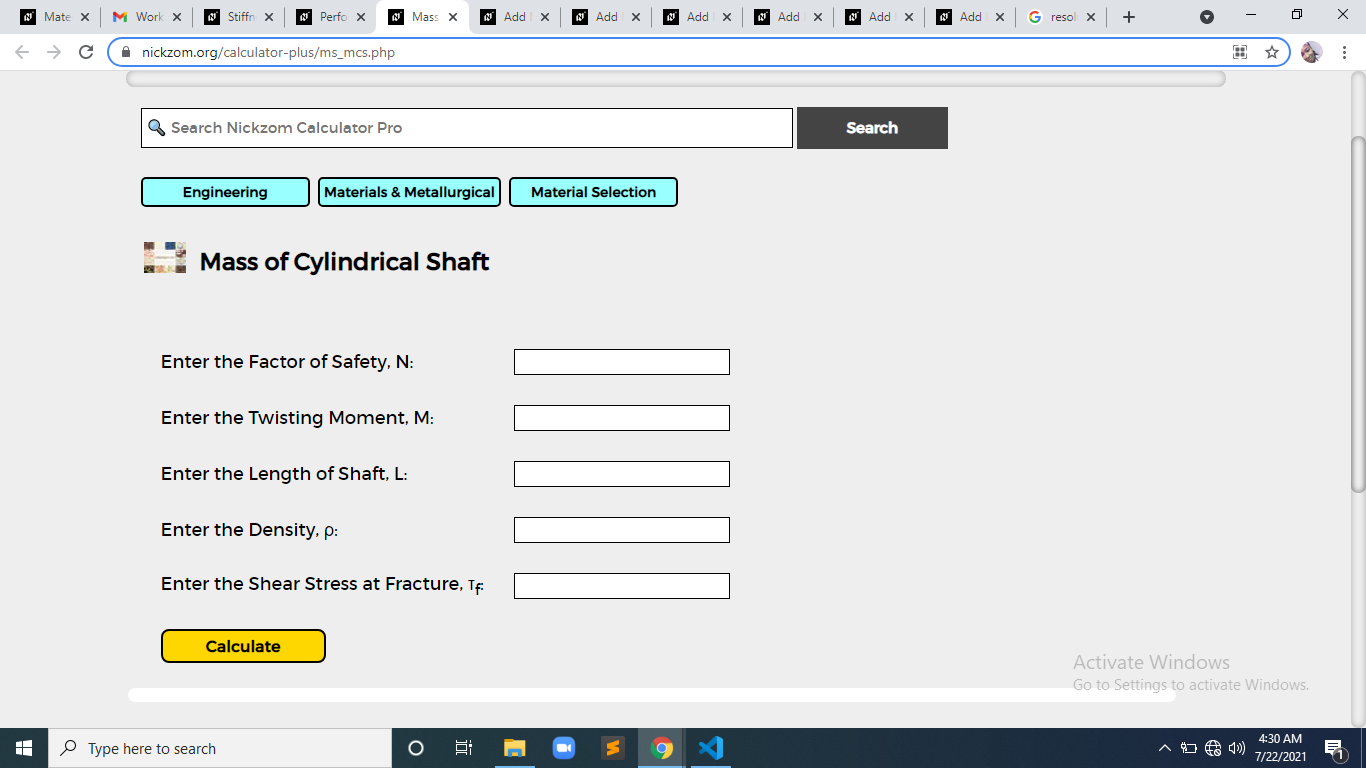Now, enter the values appropriately and accordingly for the parameters as required by the Factor of Safety (N) is 4, Twisting Moment (M) is 2, Length of Shaft (L) is 7, Density (ρ) is 6 and Shear Stress at Fracture (τf) is 10.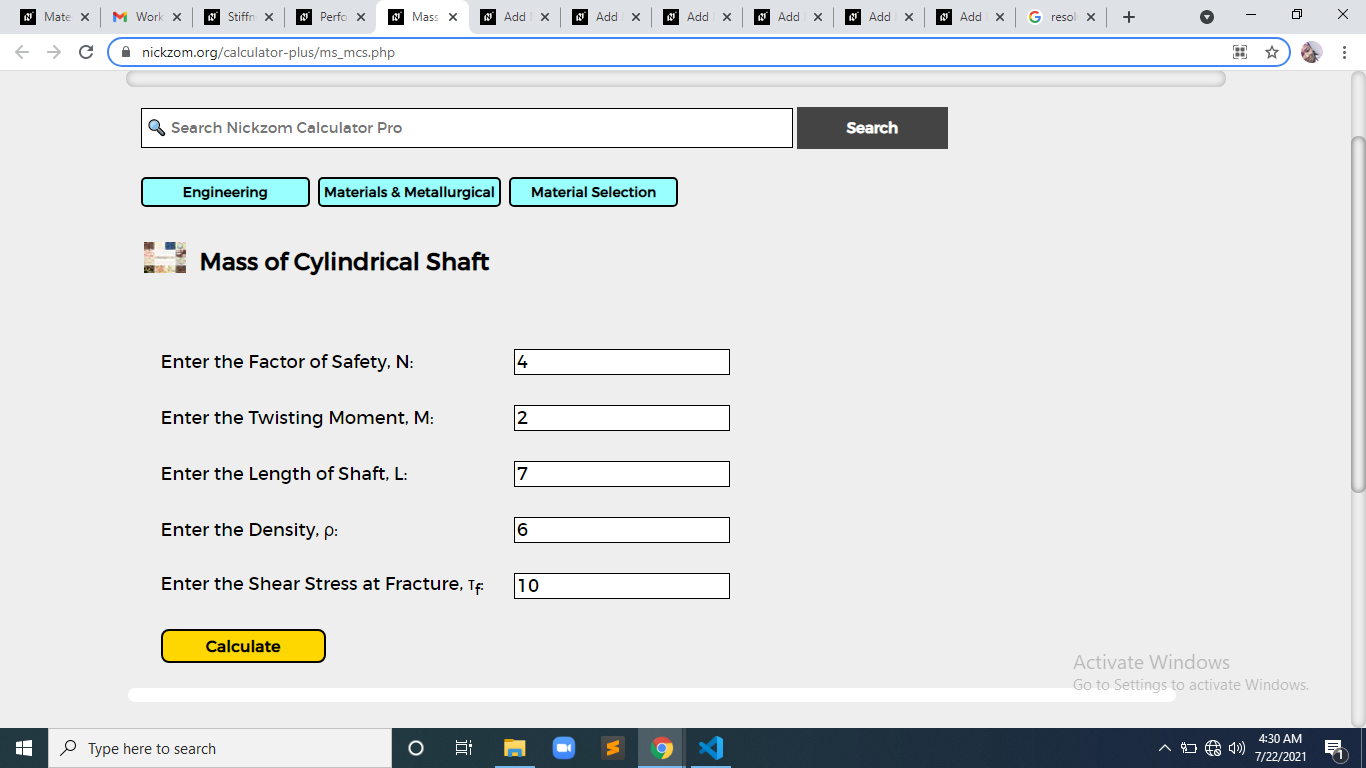Finally, Click on Calculate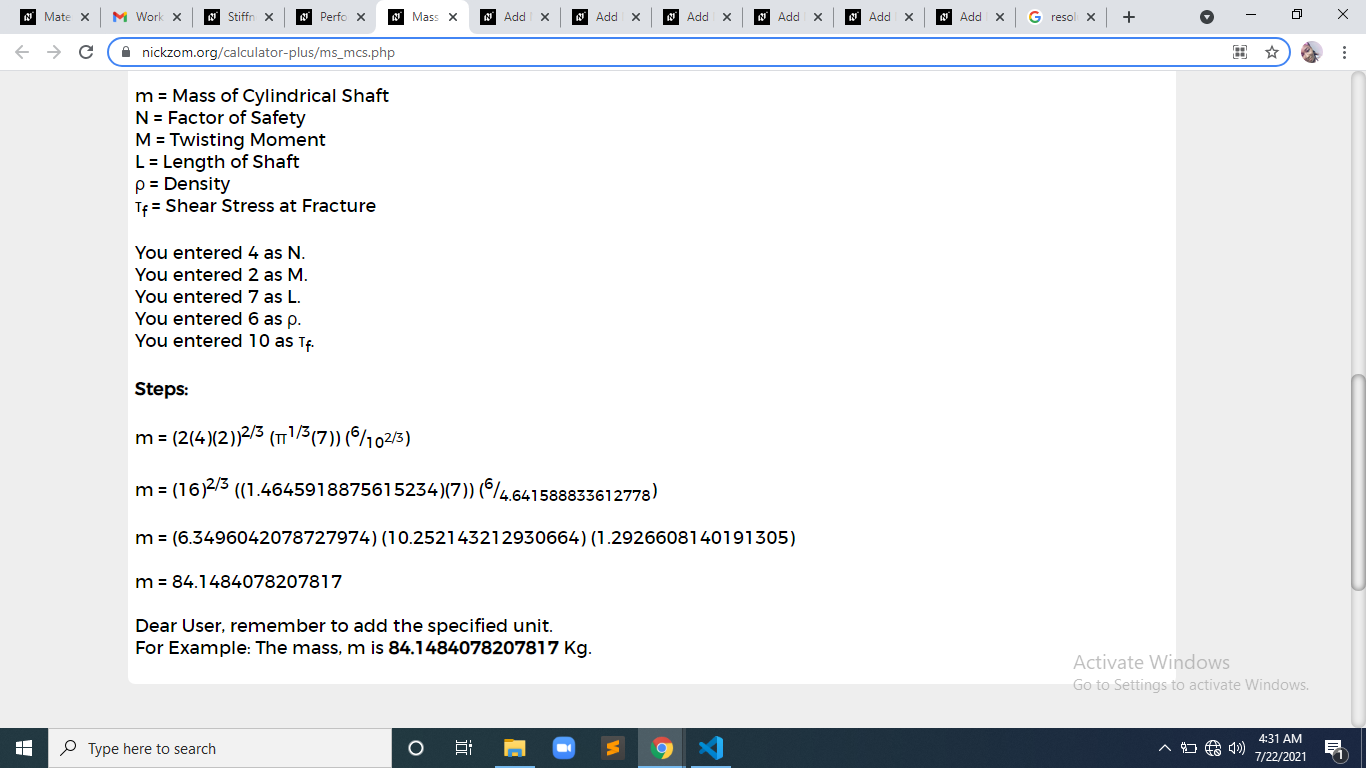As you can see from the screenshot above, Nickzom Calculator– The Calculator Encyclopedia solves for the mass of cylindrical shaft and presents the formula, workings and steps too.